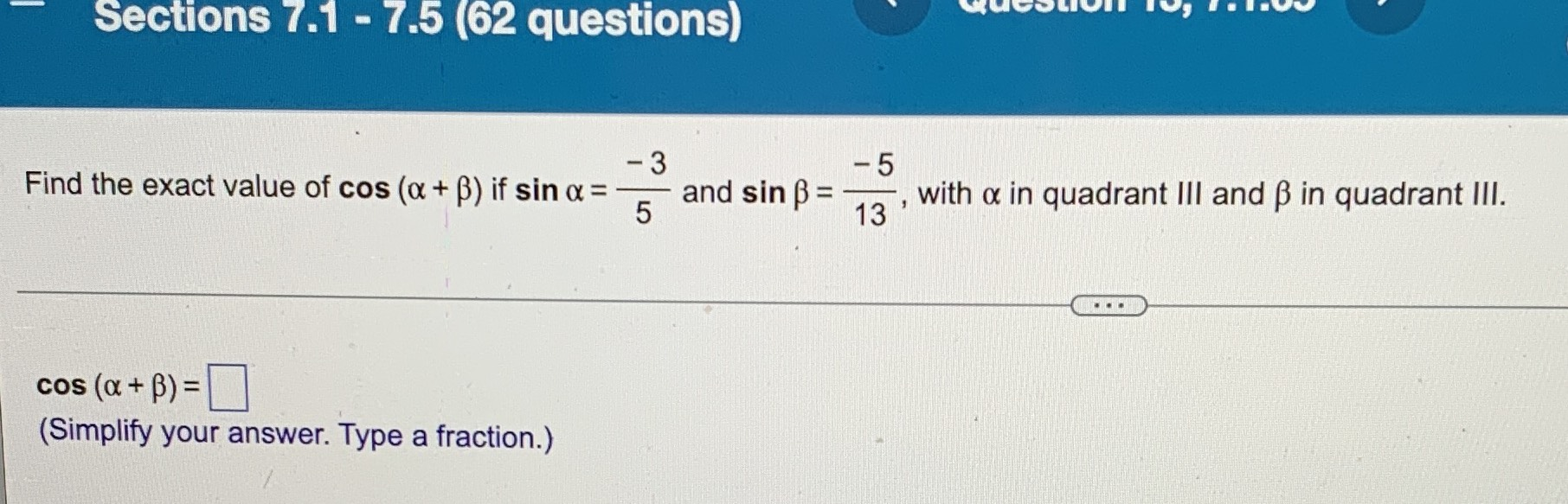### Still have math questions?

Trigonometry
QuestionFind the exact value of $$\cos ( \alpha + \beta )$$ if $$\sin \alpha = \frac { - 3 } { 5 }$$ and $$\sin \beta = \frac { - 5 } { 13 } ,$$ with $$\alpha$$ in quadrant III and $$\beta$$ in quadrant III.

$$\cos ( \alpha + \beta ) = \square$$

$$cos(\alpha + \beta )= cos\alpha cos\beta - sin\alpha sin\beta = \frac{33}{65}$$